GFG App
Open AppBrowser
Continue

# Saving Graphs as Files in R

In this article, we are going to learn how to save graphs to files in R programming language.

## Introduction

Graphs are descriptive R objects which facilitate the visual representation of data in R. The data points become more understandable and readable when expressed in the form of plots. The plots can also be saved within the local directory. They can then be accessed without opening the R editor.

## Saving graph as a pdf object

The graph can be saved as a pdf object in the local working space using pdf() function. The directory followed by the .pdf extension name is supplied as an input argument to the pdf() function.

Syntax: pdf(pdf-path)

Arguments :

pdf-path – Path of folder location where pdf file to be stored.

## R

 `# Declaring a data frame ` `data_frame <- ``data.frame``(col1=``c``(1: 5), ` `          ``col2=``c``(20, 32, 12, 57, 33)) ` ` `  `# Printing the data frame ` `print``(``"Data Frame"``) ` `print``(data_frame) ` ` `  `# Saving in pdf format ` `pdf``(``"/Users/mallikagupta/Desktop/saving_pdf.pdf"``) ` ` `  `# Plotting barplot of the data in  ` `# blue color ` `barplot``(data_frame\$col2, col=``"blue"``) ` ` `  `# shutting off the current process ` `dev.off``() `

Output:

``` "Data Frame"
col1 col2
1 1 20
2 2 32
3 3 12
4 4 57
5 5 33```

In the above code, we are creating a dataframe that is used to make a bar graph which is then saved as a pdf file using pdf() function. Plotting the bar chart using barplot() function giving the color of column blue as a parameter. The processing of the current cycle on the device can then be closed by dev.off() method.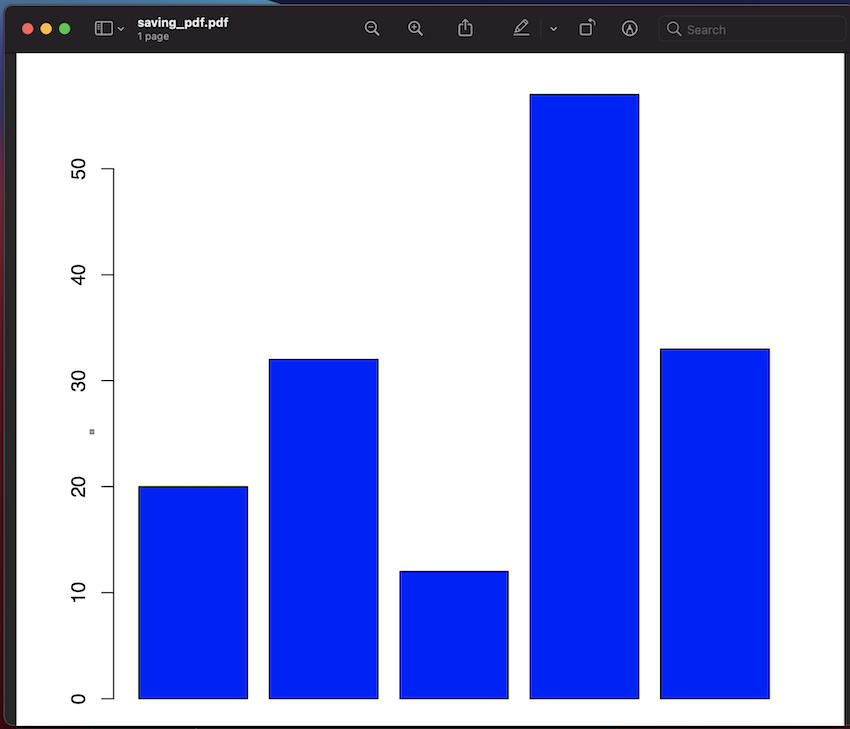## Saving graph as a PNG image

The graph can be saved as a png image object in the local working space. The directory followed by the .png extension name with the path of location supplied as an input argument to the png() function. The graph is plotted using the barplot() method, which takes vector of values as input. The processing of the current cycle on the device can then be closed by dev.off() method.

Syntax: png(png-path)

Arguments :

png-path – Path of folder location where pdf file to be stored.

## R

 `# Declaring a data frame ` `data_frame <- ``data.frame``(col1=``c``(1: 5), ` `           ``col2=``c``(20, 32, 12, 57, 33)) ` ` `  `# Printing the data frame ` `print``(``"Data Frame"``) ` `print``(data_frame) ` ` `  `# Saving in pdf format ` `png``(``"/Users/mallikagupta/Desktop/saving_png.png"``) ` ` `  `# Plotting barplot of the data ` `barplot``(data_frame\$col2) ` `dev.off``() `

Output:

``` "Data Frame"
col1 col2
1 1 20
2 2 32
3 3 12
4 4 57
5 5 33```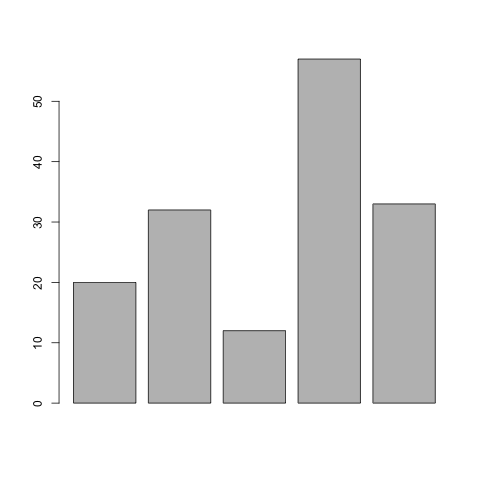## Saving graph as a .JPEG image

The graph can be saved as a jpeg image object in the local working space.  The directory followed by the .jpeg extension name with path of location supplied as an input argument to the jpeg function. The graph is plotted using the barplot() method, which takes as input the vector of values. The processing of the current cycle on the device can then be closed by dev.off() method.

Syntax: jpeg(jpeg-path)

Arguments :

jpeg-path – Path of folder location where pdf file to be stored.

## R

 `# declaring a data frame ` `data_frame <- ``data.frame``(col1=``c``(1: 5), ` `             ``col2=``c``(20, 32, 12, 57, 33) ` `             ``) ` ` `  `# printing the data frame ` `print``(``"Data Frame"``) ` `print``(data_frame) ` ` `  `# saving in jpeg format ` `jpeg``(``"/Users/mallikagupta/Desktop/saving_jpeg.jpeg"``) ` ` `  `# plotting barplot of the data ` `barplot``(data_frame\$col2) ` `dev.off``() `

Output:

``` "Data Frame"
col1 col2
1 1 20
2 2 32
3 3 12
4 4 57
5 5 33```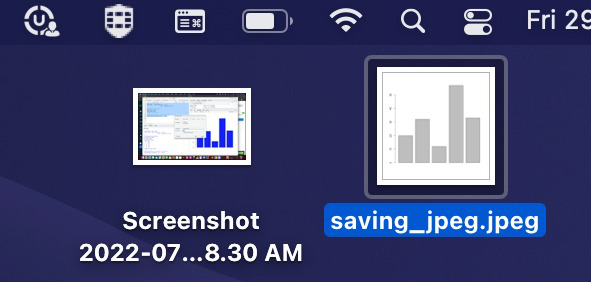Saved files of different formats## Exporting graph on own local device

The graph plotted can be then exported to our own device by clicking the “Export” icon above the image in case you are using the R studio editor. The following image shows the export icon and the options available on opening it :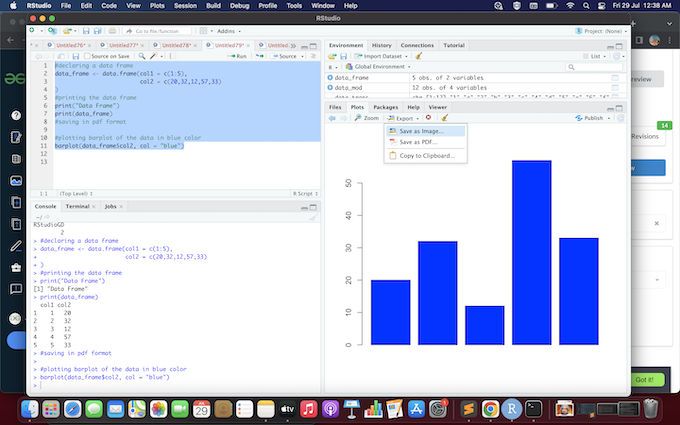If you click on “Save as Image” the following popup opens :If you click on “Save as PDF” the following popup opens and after selecting the directory click on save. It will be saved at the selected location: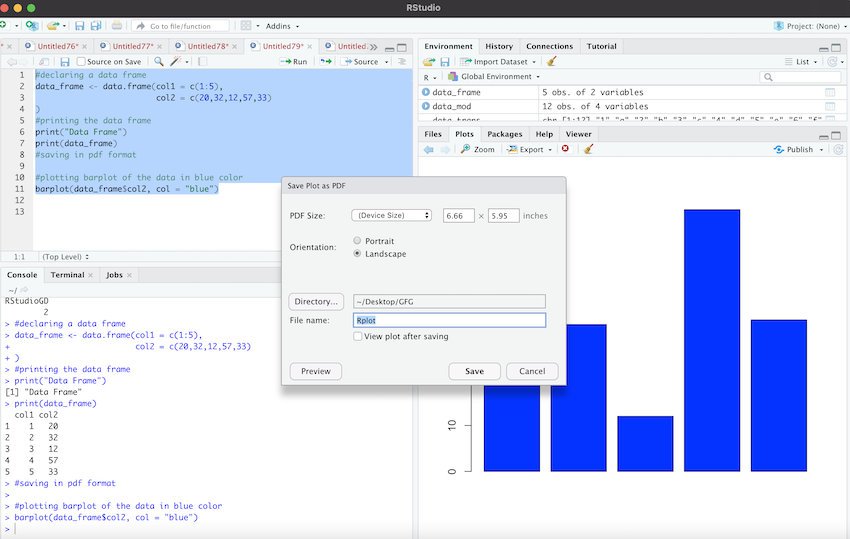My Personal Notes arrow_drop_up